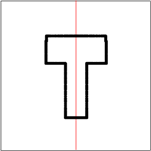Lines of symmetry
What am I?
Rotation
Reflection
100

How many lines of symmetry does a circle have?

Infinite

100

I am the only triangle that has no line of symmetry. What am I?

A scalene triangle

100

Name a letter that has 180-degree rotational symmetry.

S, X, Z, O, N, I, or H

100

What is reflective symmetry?

When one half of an object is the reflection of the other half

200

Which triangle has no lines of symmetry?

A scalene triangle

200

Two shapes that are the same shape but have different sizes are _____?

Congruent

200

How many orders of rotational symmetry does an equilateral triangle have?

3

200

Does a rectangle have reflection symmetry?

Yes

300

How do you know how many lines of symmetry a regular shape has?

The number of vertices

300

What is the property of a figure in which a line can divide the figure into two congruent parts?

Symmetry

300

Does a 5 point star have rotational symmetry?

Yes

300

How do you determine if something has reflective symmetry?

The shape can be divided equally in half reflectively

400

How many lines of symmetry does a regular polygon have?

5

400

I have 4 sides and 4 lines of symmetry. What am I?

A square

400

What is the order of rotational symmetry of a parallelogram?

2

400

Is the line a line of reflectional symmetryYes

500

How many lines of symmetry does a regular hexagon have?

6

500

I am a shape that has 5 sides, 5 lines of symmetry, and 5 vertices. What am I?

A regular polygon

500

Which of the following letters has rotational symmetry? E X T R A

Only X

500

I the line showing reflectional symmetry?No

Click to zoom